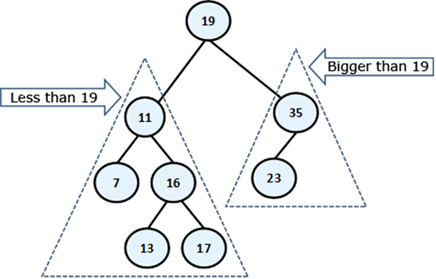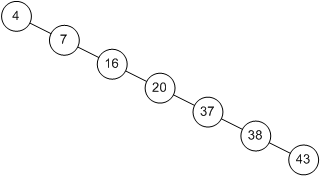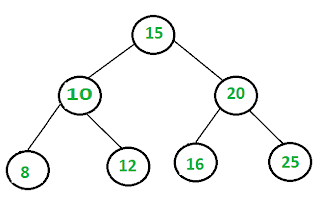Welcome to Lesson 10!Learning ObjectivesBy the end of today's class, you should know...How is a Binary Search Tree (BST) different from a Binary Tree?What is the BST Property?Why is the BST a recursive structure? How do you implement insertion for a BST?Why do you need both a helper and wrapper method when you implement the recursive BST methods?What is the Big-O run-time of insertion, search and removal operations on a BST?1. The Binary Search Tree ADT1.1. Defining a BSTA Binary Search Tree (BST) is a binary tree for which the following property is true for all nodes:The BST Property:1. Every element in node n's left subtree is less than or equal to the element in n.2. Every element in n's right subtree is greater than the element in node n.*(From the Michael Main textbook)The following tree is a BST. Verify that the above property is true for this tree.Image source.1.2. BST: A Recursive StructureRemember that the root node is linked to the root nodes of its left and right subtrees. These root nodes are in turned linked to the root nodes of other subtrees.BSTs are recursive structures because the BST property must hold true for all nodes in the tree and their subtrees.Examine the tree above. Do all subtrees meet the requirements for a BST?Due to the recursive nature of BSTs, we are able to implement most BST operations recursively.1.3. BST Operations: InsertionThe BST operations take advantage of the recursive nature of the BST.To get a better understanding of the insertion process for a BST, let's look at an example.Imagine that we are trying to insert 4 into the BST depicted in the image below.At each root node of a subtree, we need to make a decision. Do we go left? Do we go right? Or if the node is null, we should insert where we are.Image source.Thus, our process is recursive, as we are making the same decision at each node (i.e. root of a subtree).1.4. Reflection ActivityInsert the following values into a BST in the given order: 5 3 6 9 0 2 7 12 4How does the tree change if you swap the order of 5 and 9:  9 3 6 5 0 2 7 12 41.5. Insertion PseudocodeThe users of our BST class do not have direct access to any of the nodes in the BST or their data or links.However, the fact that we must recursively pass in a Node parameter (the root of the subtree) requires that we use both a helper and wrapper method in the implementation of this method. The purpose of the wrapper method is to provide a method that can be called outside of the BST class. This wrapper will in turn call the helper.The purpose of the helper method is to implement the recursive insertion algorithm. It will do most of the work of insertion.Note that the insertion of duplicates can pose a problem.Some implementations of BST insertion do not allow for duplicates*With our implementation, duplicates may cause us to amend slightly the BST property to state that all elements in the right subtree must be greater than or equal to the parent.Here is the code for the public wrapper method:public void insert(T data) {        if (root == null) {            root = new Node(data);        } else {            insert(data, root);        }    }Here is the pseudocode for the private helper method:Pseudocode for insert(node, value)1. Check if the value to insert is smaller than or equal to the data stored at node.1a. If this is true1aa. Check if node's left is null1aaa. If this is true, insert the value at node's left1b. If the node's left is not NULL1bb.recursively call the insert method passing it node's left and value2. Otherwise2a. Check if the node's right is null2aa. If this is true, insert the value at node's right2b. If the node's right is not null2bb. recursively call the insert method passing it node's right and value2. Analyzing the Efficiency of BST Operations2.1. Introduction to Run-time Analysis on Binary Search Tree OperationsThe Big-O run-time of the BST operations is closely allied to whether or not the tree is balanced.Recall that there are several definitions of balanced.In this class, we consider a balanced tree to be one that for all nodes in the tree, the following equation is true:| heightleft - heightright| <= 1 Why is this?Consider a binary search tree like this one:Now think about searching this tree for a specific value.This binary search tree is no different than a linked list.Is search efficient on a linked list? We should have seen in Lab 4 that searching a linked list is not efficient.Because linked lists do not offer random access of their elements, binary search is not an efficient algorithm for a linked list.For binary search trees organized like the one above, search also takes O(n) time, as does insertion and removal.BST Operations Worst Case: O(n)We are going to see with a more balanced binary search trees, operations such as search, insert and remove are possible in O (log n) time, just like binary search on an array.BST Operations Average Case: O(log2 n)Why are these operations O(log2 n) for a BST?Consider search. In the image below, we are searching for the value 4 to determine if it is in the tree:As in binary search, we continually divide the search space in half until there is only one element:n -> n / 2 -> n / 4 -> n/ 8... -> n/ 2k = 1Multiply both sides by 2kn = 2kThen take the log of both sides:log2 (n) = kThis leaves us with O(log2 n).Let's walk through an example to verify this works on a balanced BST. What happens if we search for the value 12?Image source.Insertion and removal have the same average runtime as search, as both take advantage of the organization of data within the tree. However, given a BST, there is no guarantee that the tree will be balanced, or means of enforcing balance -- hence the worst case of O(n).2.2. Comparison of Arrays, Linked Lists and Binary Search TreesLet's compare the average case Big-O run-times of Arrays and Linked Lists to those of the BST when performing 3 common ADT operations:Array            Linked List            Binary Search Tree            Search                O(log n)                O(n)                    O(log n)Insertion             O(n)                     O(n)                    O(log n)Deletion              O(n)                     O(n)                    O(log n)2.3. Animated BST Efficiency compared to a Linked List (although it says array)image sourceWrap UpAnswer the review questions on Canvas for today's lesson